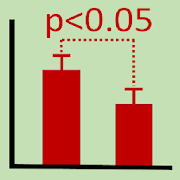Stats Tester, Easy statistics calculatorEveryone
89
## Important information for Samsung users ##

On some Samsung GALAXY models, there may be no decimal, minus or space key on the numeric keyboard. In that case, please install Google Keyboard from the Play Store and try it as the default keyboard. This also solves similar problems in other calculation apps.

By using Stats Tester, you can do statistics calculation and analysis by an easy operation. This app has the following features.

+ Each statistics method has useful [Help] and [Example] buttons.

+ You can put data into the input-window by using a keyboard or by pasting data from this app (other statistics methods) or other apps (e.g. text editors and Google Sheet).

+ Since one input-window([A]..[D]) accepts entering of many numbers, it is easy to edit entered data.

+ Except for the frequency data, the maximum number of samples that can be processed is 100/group. Chi-square and Fisher exact tests accept up to frequency of 1,000,000.

+ When entering a lot of data, it is convenient to paste data from Google Sheets, etc. Original and result data can be saved to the same file by using copy/paste functions.

+ Result data can be sent to mailers or saved to clouds(Google Drive, OneNote etc) by [Share] button. Data on the output-window can be copied by [Copy Results to ClipBoard] button. ([Input-window] has own copy/paste functions.)

+ Entered data and results calculated by each method are memorized until the next use. This function is a feature of this app and it is very convenient. (Since data are saved in special memory that can only be accessed with this app, the data can not be read or written by other apps. [All Clear] button of option-menu(3 dot) on the first screen erases saved all data.)

+ This app can be used for basic statistics, biostatistics and medical statistics, etc. It can be also used to study statistical method by using textbooks.

+ With Chromebooks, at least two Android apps can be used simultaneously, making collaboration with other apps easier.

## Acknowledgment: The AChartEngine library distributed under the Apache 2.0 license was utilized to make chart.
https://github.com/ddanny/achartengine

* Parametric statistics:

1. Mean, SEM and SD, etc
- Arithmetic, Geometric and Harmonic means
- Standard Error, Standard Deviation
- Confidence interval
- Sum
- Sum of Square
- Variance
- Skewness
- Kurtosis

2. SD-SEM Conversion
(Converter between Standard Deviation and Standard Error)

3. One-sample t-test

4. Paired t-test

5. t-test (Student and Welch)
- F-test to compare 2 variances
- t-test(Student and Welch)

6. F-test for equality of 2 variances
- Mean and SD
- F-test

7. Levene test for homogeneity of variance
(n >=3 groups)

8. One-way ANOVA

9. Multiple t-test (with Bonferroni's correction)

10. Correlation and Regression
- Correlation coefficient
- Regression coefficient
- Coefficient of determination
- Test for correlation coefficient

* Nonparametric statistics:

1. Median, Range and Quartiles, etc
- Minimum, First, second and third Quartiles, Maximum, Quartile deviation

2. Mann-Whitney's U test

3. Median test

4. Kruskal-Wallis test and multiple comparisons

5. Sign Test

6. Wilcoxon's signed-rank sum test
(paired 2 groups)

7. Chi-square test (Goodness of Fit)

8. Chi-square test(Independence, 2x2)

9. Chi-square test(Independence, mxn)

10. McNemar's test (paired case)

11. Fisher's exact test

12. Spearman rank correlation coefficient
Collapse

Review Policy
4.3
89 total
5
4
3
2
1

Collapse

Updated
October 17, 2018
Size
2.2M
Installs
10,000+
Current Version
3.1.2
Requires Android
4.1 and up
Content Rating
Everyone
Permissions
Offered By
BMP group